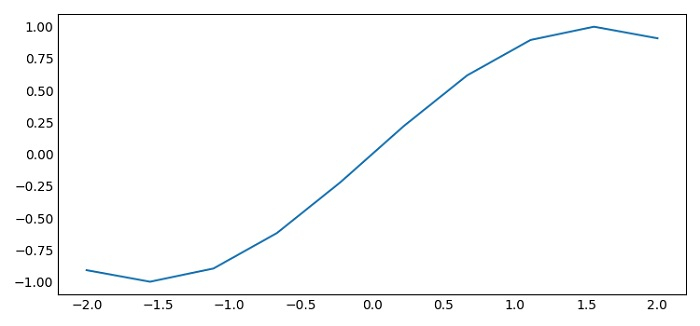# Turn off the left/bottom axis tick marks in Matplotlib

To turn off the left or bottom axis tick marks in matplotlib, we can use length=0 for the axes.

## Steps

• Set the figure size and adjust the padding between and around the subplots.

• Create x and y data points using numpy.

• Plot the x and y data points using plot() method.

• Use tick_params() method with length=0.

• To display the figure, use show() method.

## Example

import numpy as np
import matplotlib.pyplot as plt

plt.rcParams["figure.figsize"] = [7.50, 3.50]
plt.rcParams["figure.autolayout"] = True

x = np.linspace(-2, 2, 10)
y = np.sin(x)

plt.plot(x, y)
plt.tick_params(axis='both', which='both', length=0)

plt.show()

## Output